# How to Count Dates of Given Year in Excel

This post will guide you how to count Dates of a certain year in the range of dates using a formula in Excel 2013/2016 or Excel office 365. How do I count dates by a given year in Excel. And you may be want to count the cells where dates are from a particular year. It should be similar with the previous post that how to count dates by day of week. And we can use the SUMPRODUCT function and the YEAR function to build a formula to count the date values where the date belongs the specific given year.

## Count Dates of Given Year Through Formula

Assuming that you may be want to count dates of given year in date range B1:B6. You can use the below formula based on the SUMPRODUCT function and the YEAR function, like this:

=SUMPRODUCT(–(YEAR(\$B\$1:\$B\$6)=D2))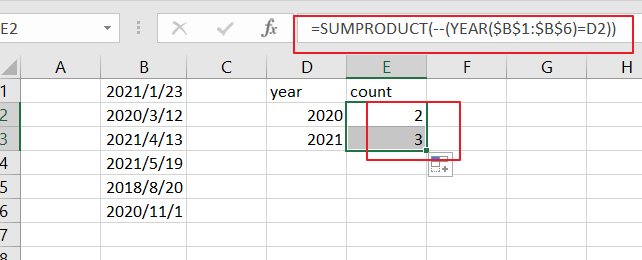Note: you need to change date range as you need. And changing year value given as cell reference.

LETS SEE THAT HOW THIS FORMULA WORKS

=YEAR(B1：B6)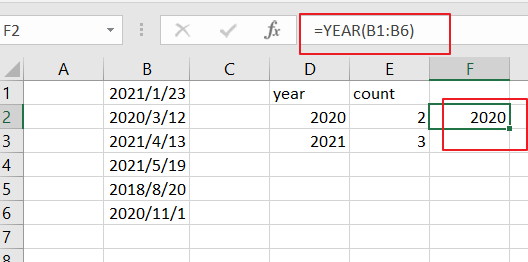The YEAR function will extract the year value from the given data range. And it returns an array result like this:

{2021;2020;2021;2021;2018;2020}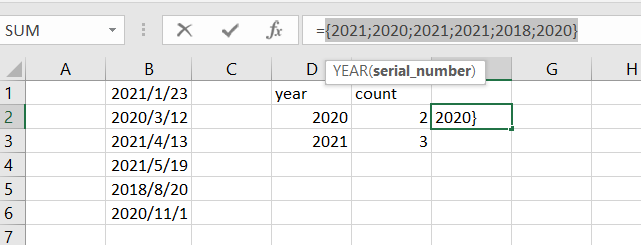=YEAR(B1：B6)=D2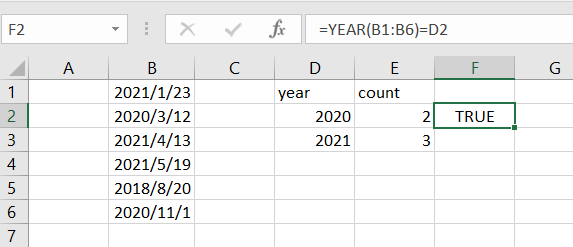Then the array results returned by YEAR formula are compared to the given year value, and returns another array result of TRUE and FALSE values like this:

{FALSE;TRUE;FALSE;FALSE;FALSE;TRUE}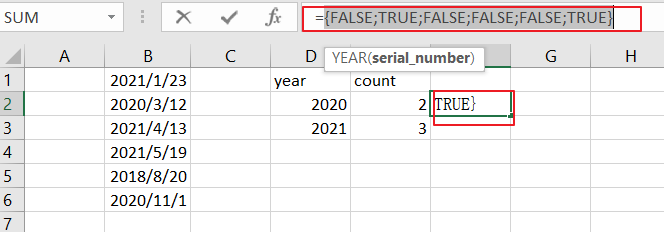=–(YEAR(B1:B6)=D2)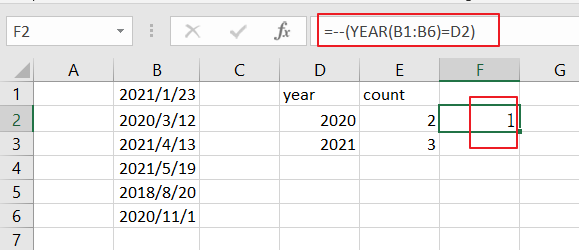You still need to convert the TRUE or FALSE values to 1 and 0 values using the double negative operator. And returns the below array result:

{1;0;0;0;0;0}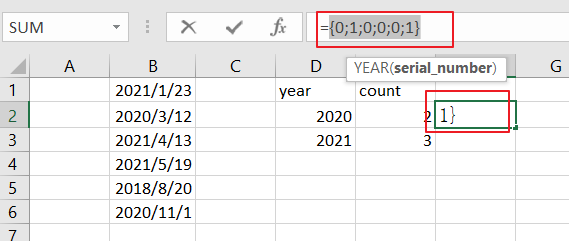The SUMPRODUCT function just sums all values of an array returned by the above formula.

You can see that the total date matches of the year 2020 comes out to be 2. And it means that there are 2 date values where the year value equals to 2020.

### Related Functions

• Excel SUMPRODUCT function
The Excel SUMPRODUCT function multiplies corresponding components in the given one or more arrays or ranges, and returns the sum of those products. The syntax of the SUMPRODUCT function is as below:= SUMPRODUCT (array1,[array2],…)…
• Excel YEAR function
The Excel YEAR function returns a four-digit year from a given date value, the year is returned as an integer ranging from 1900 to 9999. The syntax of the YEAR function is as below:=YEAR (serial_number)…
Related Posts

Add Months To Date In Excel

It is important to adjust time periods when performing financial modeling. In Excel, you can use the MONTH function to add a specific number of months to a date. The MONTH function takes one argument: the number of months you ...

Add Days to Date in MS Excel

If you are an vavid MS Excel user, then you might have come across situations where you need to add the same or different days into the particular date, and if you have done this task manually, then let me ...

Filter Data By Date Field

The use of Microsoft Excel's Format Cells and Auto Filter option is a great way to filter data by year or Month. This article will discuss how to filter by date field using Filter function and Format cells in Microsoft ...

How to Sum Values Based on Month and Year in Excel

We often do some summary or statistic at the end of one month or one year. In these summary tables, there are at least two columns, one column records the date, and the other column records the sales or product ...

How to Convert Date & Time Format to Date in Excel

Sometimes we want to convert date and time format to date only format in excel for example convert 01/29/2019 06:51:03 to 01/29/2019, we can convert format by Formula or Format Settings. The two ways are easy to learn, so you ...

How to Extract Year from Date & Time Format in Excel

Sometimes we want to get Year information from date and time format to show the Year only in excel. For example convert 01/29/2019 06:51:03 to 2019, we can get Year information by Formula or Format Settings. The two ways are ...

How to Calculate Remaining Days in a Month or Year in Excel

This post will guide you how to calculate remaining days in a given month or year in Excel. How do I calculate the number of days left in a month or year using a formula in Excel 2013/2016. Calculate Remaining ...

How to Split Date into Day, Month and Year in Excel

This post will guide you how to split date into separate day, month and year in excel. How do I quickly split date as Day, Month and Year using Formulas or Text to Columns feature in Excel. Split Date into ...

How to sort Dates by Month and Day Only in Excel

This post will guide you how to sort dates or birthdays by month or day only in Excel. How do I sort dates by Year or Month or Day only with a formula in Excel 2013/2016. How to use the ...

How to Add the Current Month or Year in a Cell in Excel

This post will guide you how to add the current month or year into a cell or header or footer in your worksheet. How do I insert the current date or time in a cell with a formula in Excel. ...

Sidebar